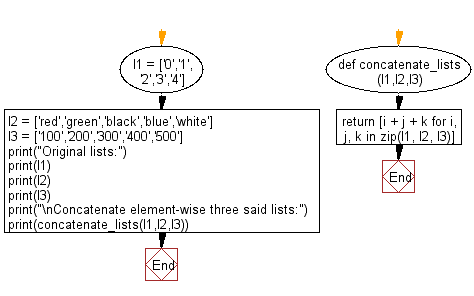﻿ Python: Concatenate element-wise three given lists - w3resource# Python: Concatenate element-wise three given lists

## Python List: Exercise - 171 with Solution

Write a Python program to concatenate element-wise three given lists.

Sample Solution:

Python Code:

``````def concatenate_lists(l1,l2,l3):
return [i + j + k for i, j, k in zip(l1, l2, l3)]

l1 = ['0','1','2','3','4']
l2 = ['red','green','black','blue','white']
l3 = ['100','200','300','400','500']

print("Original lists:")
print(l1)
print(l2)
print(l3)
print("\nConcatenate element-wise three said lists:")
print(concatenate_lists(l1,l2,l3))
```
```

Sample Output:

```Original lists:
['0', '1', '2', '3', '4']
['red', 'green', 'black', 'blue', 'white']
['100', '200', '300', '400', '500']

Concatenate element-wise three said lists:
['0red100', '1green200', '2black300', '3blue400', '4white500']
```

Pictorial Presentation:Flowchart:## Visualize Python code execution:

The following tool visualize what the computer is doing step-by-step as it executes the said program:

Python Code Editor:

Have another way to solve this solution? Contribute your code (and comments) through Disqus.

What is the difficulty level of this exercise?

Test your Python skills with w3resource's quiz

﻿

## Python: Tips of the Day

Floor Division:

When we speak of division we normally mean (/) float division operator, this will give a precise result in float format with decimals.

For a rounded integer result there is (//) floor division operator in Python. Floor division will only give integer results that are round numbers.

```print(1000 // 300)
print(1000 / 300)```

Output:

```3
3.3333333333333335```